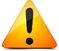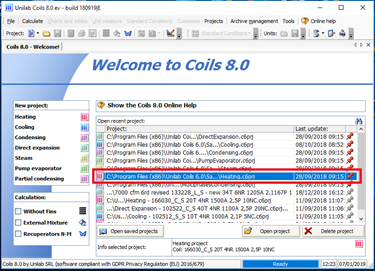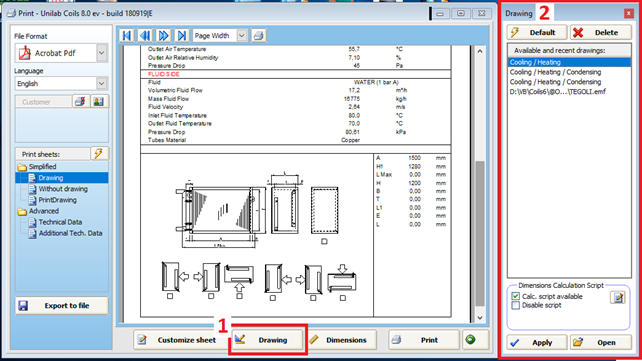For a complete understanding of the paragraph it is useful to have a minimum knowledge of VBS scripts

STEP 1

Load/Create a coil project.STEP 2

Calculate and print the project with drawingSTEP 3

Press the button “Drawing” to display the form “Drawing”STEP 4

Edit the notepad to modify the script to calculate the dimensionsSTEP 5 (Example)

The script of the STEP 4 calculates the H1 = H + 80 mm